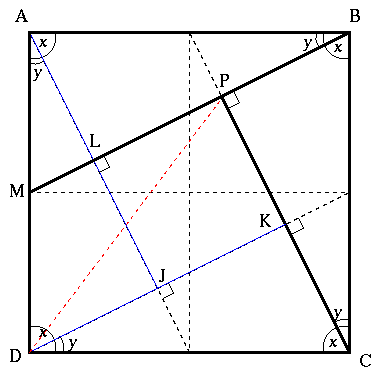#### You may also like### Fitting In

The largest square which fits into a circle is ABCD and EFGH is a square with G and H on the line CD and E and F on the circumference of the circle. Show that AB = 5EF. Similarly the largest equilateral triangle which fits into a circle is LMN and PQR is an equilateral triangle with P and Q on the line LM and R on the circumference of the circle. Show that LM = 3PQ### Look Before You Leap

Can you spot a cunning way to work out the missing length?### Triangle Midpoints

You are only given the three midpoints of the sides of a triangle. How can you construct the original triangle?

# Two Triangles in a Square

##### Age 14 to 16 Challenge Level:

You may like to ponder the fact that, looking at David's diagram below, the square $LPKJ$ is 1/5th of the area of the square $ABCD$.

The following solution came from David (Madras College).Label angles $x$ and $y$, where $x + y = 90^{\circ}$, $\angle MBC = x$ and $\angle ABM = y$

A line is drawn from point $A$ to point $L$ so that $\angle ALB = 90^{\circ}$

Triangles $ABL$ and $BCP$ are congruent because they have equal angles and $AB = BC$. Similarly triangles $CDK$ and $DAJ$ can be proven congruent with $ABL$ and $BCP$ forming a "camera shutter'' shape.

Triangle $ABM$ is similar to the four congruent triangles (with angles $x$, $y$ and $90^{\circ}$) and therefore the proportions are the same.

Because $AB = 2AM$ we have $PC = 2PB$ and then, as $KC = PB$, we have $PC = 2KC$, so $K$ is the midpoint of the line $PC$.

When triangle $CDK$ is reflected along the line $DK$, the line $DP$ is the reflection of the line $DC$ and they are therefore the same length.